cancel
Showing results for
Did you mean:

Fabric is Generally Available. Browse Fabric Presentations. Work towards your Fabric certification with the Cloud Skills Challenge.Regular Visitor

## Numeric range dropdown filter

Hi

I have an excel dataset as underlying data. It is housing data, so for each row i have a column saying how many rooms the apartment have. See the image below for the column and values.The stakeholders want to have two filters in the report. One saying "Room from" and the other "Room to". So basically they want a numeric range slicer, but as a dropdown list filter, something like the image below:The problem is that if i choose From = 2 and To = 5 it does not filter the data from 2 TO 5, it only shows data with room = 2 AND room = 5, as expected. I want the data to show the range between the to options.

I believe there must be a work around on this issue with nested if statements or a function?

I tried to solve it by creating two seperate tables with unique values containing all possible rooms(picture below), one "from" and one "to". My next idea was to find a function saying when choosing 2 from the FROM table/filter and 5 from the TO table/slicer, the data filters everyting from 2 to 5 room apartments.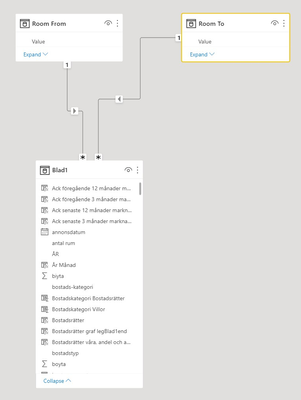Has anyone faced this issue before and can you guys help me out with this?

20 REPLIES 20Community Support

Hi @arvin ,

You will need to create two separate tables as slicer (from and to) and make sure there's no relationship between these two table and the fact table.

Create a measure, let's call it [measure1], to get the number of rooms (which you will use to compare with the number selected in the slicers). You could add it to matrix to check if it gets the correct result then remove from visual.

Then create the second measure.

Measure2 =
var _min = selectedvalue(from_slicer[from])
var _max = selectedvalue(to_slicer[to])
return
IF([measure1]>=_min&&[measure1]<=_max,1,0)

At last, add the measure to visual filter and set value = 1.

If it still doesn't work, please show some sample data and expected result so that we could test the formula.

Best Regards,

Jay

Community Support Team _ Jay
If this post helps, then please consider Accept it as the solution
to help the other members find it.Regular Visitor

Hi @v-jayw-msft
How do i get the number of rooms for measure 1? VALUES?Community Support

Hi @arvin ,

That is based on your data and requirement. I don't know what your data looks like and I don't know what result you want. Share the sample data without confidential information and the expected result, so that we could decide to use sum() or max() or min() or other function to get it.

Best Regards,

Jay

Community Support Team _ Jay
If this post helps, then please consider Accept it as the solution
to help the other members find it.Regular Visitor

Hi @v-jayw-msft

So, I have an excel dataset of housing data. In my report i have a matrix, a card visual and graphs.

As for now, i use the "room" column in a slicer to filter the visuals on room.

An apartment can have 1 room or 15 rooms, and everything between.

The stakeholder wants to filter the visuals with a "From Room" and "To Room" dropdrown filter.

I want the reuslt to be like this:  if i choose From = 2 and To = 5, i will see housing data from 2-5 room apartments.

This is the "room" column:I have a card visual, a matrix and a graph. I want all the visuals to adjust when i select for example rooms between 2-5(see picture below).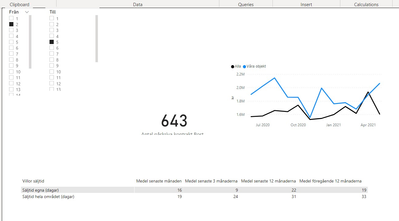Super User

Hi,

Here is one way to do this:

First I created to parameter for slicing the values. Then I created a filter measure:

RoomsFilter =
var _rooms = max(RoomsToFrom[Rooms]) return

IF(and(_rooms<=[RoomsTo Value],_rooms>=[RoomsFrom Value]) ,1,0)

Now I just applied Filter measure to my table: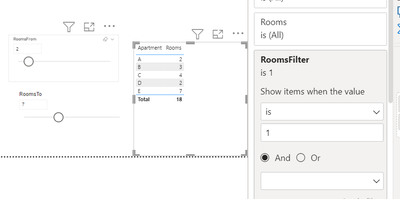By using these steps the behaviour you described can be achieved.

I hope this post helps to solve your issue and if it does consider accepting it as a solution and giving the post a thumbs up!

Proud to be a Super User!Regular Visitor

Hi, I did ecatly what you said and tried to apply the measure in my matrix, but it doesnt seem to work, it worked on tables, but i have multiple matrixes like the image below and nothing happens:Super User

Hi,

It is important the filter is "Rooms filter is 1" Now you have "Rooms filter is 1 or 0" This defeats the purpose and it is likely the reason the filter is not working.

Proud to be a Super User!Regular Visitor

Sorry i sent the wrong printscreen. Here is the correct one. I have the measure = 1 and nothing shows.Super User

Hmm,

That is curious behaviour. Could you show the DAX you used?

Proud to be a Super User!Regular Visitor

It works on tables and graphs, but when i have no option selected in the parameters the data disapears, can i create the measure so that when nothing is selected, all data is showing?

It does not work on matrixes and cards, in the picture below i can't select the Rooms Filter = 1.Super User

Here is modified filter which works also when nothing is selected:

RoomsFilter =
var _rooms = max(RoomsToFrom[Rooms])
var _roomsfrom = IF([RoomsFrom Value]=BLANK(),0,[RoomsFrom Value])
var _roomsto = IF([RoomsTo Value]=BLANK(),999,[RoomsTo Value])
return

IF(and(_rooms<=_roomsto,_rooms>=_roomsfrom) ,1,0)

Proud to be a Super User!Regular Visitor
I created two parameters; Från(from) and Till(to)

The measure is below:
-Blad1[antal rum] is the column with rooms in my dataset.
RoomsFilter =
return
IF(and(_rooms<=[Till Value],_rooms>=[Från Value]) ,1,0)

I do have a measure in the matrix Values section, i dont know if that creates a problem.Super User

The filter measure seems to be correct. It is entirely possible that your other measure in matrix values causes the issue. Here is an example of that:

Impossible measure = CALCULATE(SUM(RoomsToFrom[Rooms]),RoomsToFrom[Rooms]=1)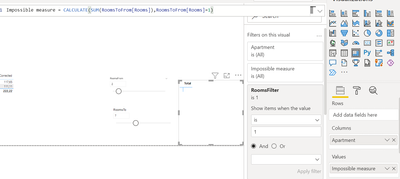To solve this you can remove the filter context from this measure, since I don't know what your measure does I can only suggest using ALL.
E.g.

Impossible measure2 = CALCULATE(SUM(RoomsToFrom[Rooms]),RoomsToFrom[Rooms]=1,ALL(RoomsToFrom))There are better ways to do this, but I would need to know what your other measure does.

Proud to be a Super User!Regular Visitor

Below you can see the calculated columns and measures that i use as cards and matrixes. The graph works perfectly with the parameter but the matrix doesnt show any data and the card and can't set the Rooms Filter to = 1.

Graph(works)
The graph works fine. I have a date column as axis, a calculated column as Legend and a measure in Values:
-Legend:

Calculated Column=
if(Table1[columnX] = "Legend1", "Legend2",
if(Table1[columnX] <> "Legend1" || Table1[columnX] = "Legend1","All"))
-Values:
Measure =
CALCULATE(AVERAGE(Table1[columnY]),Table1[columnZ] = "B")
I also have a date filter on the filters pane saying only look at data from today and 3 months back.

Card(doesnt work)
Measure =
CALCULATE(COUNT(Table1[ColumnY]),Table1[ColumnZ] = "B")
I also have a date filter on the filters pane saying only look at data from today and 3 months back.

Matrix(doesnt work)

-Rows
Calculated Column =
if(Table1[ColumnX] = "example text", "output",
if(Table1[ColumnX] <> "example text"|| Table1[ColumnX] = "example text", "output2"))

-Columns
Calculated Column =
if(Table1[Datesegment] = "1", " X",
if(Table1[Datesegment] = "2", " Y",
if(Table1[Datesegment] = "3", " Z",
if(Table1[Datesegment] = "4", "F"))))
-Values
Measure =
CALCULATE(AVERAGE(Table1[price]),Table1[category] = "V")Super User

@arvin

Card:

Normally you can't filter card with a measure. To circumvent this we can first create a table visual and place the filter there:Now convert it to a card: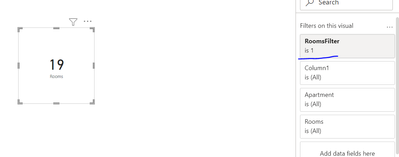As for the matrix:

Instead of nested IFs you should use
SWITCH(TRUE(),
condition1,result1,
condition2,resul2) structure.

This doesn't solve the issue but it is more readable.

My assumption is that your columns work and the issue is with the measure. You can you test this b testing the visual with this:

TestMeasure =
CALCULATE(AVERAGE(Table1[price]),Table1[category] = "V",ALL(Table1))

If this return values this should work:

Working Measure =
var cc1 = Max(Calculated Column)
var cc2 = Max(Calculated Column2) return

CALCULATE(AVERAGE(Table1[price]),Table1[category] = "V",ALL(Table1),
Table1[Calculated Column]=cc1,Table1[Calculated Column]=cc2)

Proud to be a Super User!Regular Visitor

Hi @ValtteriN

I tried the card trick and it did not work when choosing a parameter, the value is the same every time.

I also tried the test measure for the matrix, but all values in the matrix became identical and when choosing parameters they all disappeared, picture below:

TestMeasure =

CALCULATE(AVERAGE(Table1[price]),Table1[category] = "V",ALL(Table1))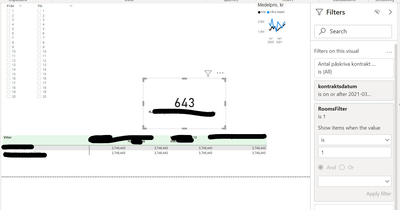Super User

Hi,
@arvin
The test measure did it job if you got some values into the matrix. Now we just need to figure out how to get the filter context to function based on this test. What values did the "working measrure" return?

Proud to be a Super User!Regular Visitor

The matrix now shows correct values with the "working measure" in the values section.
The problem is still that it does not respond accurate to the parameters, when choosing for example from = 2 and to = 4, the data in the matrix disappears.Regular Visitor

This is a example of the card measure:

Card Measure =

This is the measure that I use in the matrix:

Matrix measure =Super User

@arvin  To achieve a between behaviour, you need to use all the (From, To) values from the same table and they can't come from two different tables.

Proud to be a Super User!

New Animated Dashboard: Sales CalendarAnnouncements#### Power BI Monthly Update - November 2023

Check out the November 2023 Power BI update to learn about new features.#### The largest Power BI and Fabric virtual conference

130+ sessions, 130+ speakers, Product managers, MVPs, and experts. All about Power BI and Fabric. Attend online or watch the recordings.Top Solution Authors
Top Kudoed Authors
Users online (6,431)